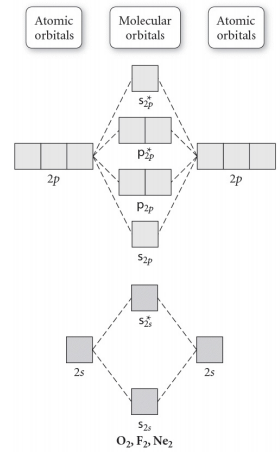# Problem: Use the molecular orbital diagram shown to determine the bond order for NO +. Is NO+ paramagnetic or diamagnetic? In molecular orbital names, s = sigma and p = pi.a. 3, paramagneticb. 2, paramagneticc. 2.5, paramagneticd. 2, diamagnetice. 3, diamagnetic

###### FREE Expert Solution

Construct the molecular orbital diagram of NOand calculate for the bond order and determine if its paramagnetic or diamagnetic

Step 1: Calculate the total number of valence electrons present.

Step 2: Draw the molecular orbital diagram.

Step 3: Determine if there's an unpaired MO (paramagnetic or diamagnetic)

Step 4: Calculate the bond order of the molecule/ion. Recall that the formula for bond order is:

90% (190 ratings)###### Problem Details

Use the molecular orbital diagram shown to determine the bond order for NO +. Is NO+ paramagnetic or diamagnetic? In molecular orbital names, s = sigma and p = pi.a. 3, paramagnetic

b. 2, paramagnetic

c. 2.5, paramagnetic

d. 2, diamagnetic

e. 3, diamagnetic